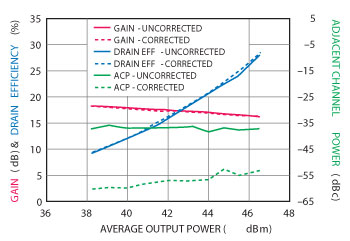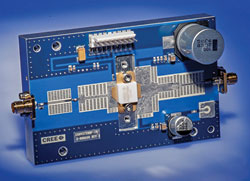With the advent of telecommunication applications requiring higher RF peak and average power levels in most of the frequency bands covering 700 MHz to almost 3 GHz, GaN HEMTs with higher operating voltages are gaining popularity. Until recently, most GaN HEMT technologies were limited to operating drain voltages of 28 V. Cree has introduced a range of 50 V GaN HEMT transistors that provide significant advantages over their 28 V siblings. They provide higher power, improved bandwidths and a larger number of parts per wafer for the same power level reducing costs and increasing volume throughput. In addition to these advantages, the new 50 V devices exhibit minimum drain-to-source breakdown voltages of 125 V providing ruggedness when operating into 10:1 output VSWRs with arbitrary phase angles.

The first devices in the 50 V family, specifically designed for cellular infrastructure applications, are the CGHV22100, 27100, 22200 and 27200. These devices operate in the 1.8 to 2.2 GHz and 2.5 to 2.7 GHz bands, with peak output power levels in excess of 100 and 200 W. A summary of the linear performance of these transistors is shown in Table 1. All four transistors are available in the 440161 and 440162 earless (pill) and eared (flange) style metal/ceramic packages. These transistors have internal input matching consisting of wire-bonds and shunt capacitors to raise the real part of the input impedance to approximately 10 ohms making PCB matching relatively simple and straightforward. The output of the transistor does not require internal matching (partially owing to the results of 50 V operation on the output load-lines). An additional advantage of this approach is that harmonic matching may also be applied at the PCB level to enhance efficiency.The internal input matching is highly reproducible owing to the employment of fully automated, programmable wire-bonders that define wire length, loop height and profile as well as automatic die placement and attachment. The design of the internal matching circuitry is provided by the use of 3D electromagnetic simulation including the transition from the inside to the outside of the package through the package ceramic ring frame.

Figure 1 shows the typical drain efficiency and adjacent channel leakage ratio (ACLR) for the CGHV22100F measured in an evaluation board at 1.8, 2 and 2.2 GHz. Typical drain efficiency at an average output power of 44 dBm (1 carrier 3GPP signal with 5 MHz channel bandwidth with a peak-to-average ratio (PAR) of 7.5 dB) is 32 percent at an ACLR of –37 dBc. Power gains are in excess of 21 dB. This compares with a power gain of 15 dB for a 28 V “equivalent” transistor such as the CGH21120F. The significant power gain increase is a direct result of operation at 50 V.Figure 1 Typical drain efficiency and ACLR vs. output power of the CGHV22100 measured in CGHV22100-TB amplifier circuit (VDS = 50 V, IDS = 0.5 A, 1c WCDMA, PAR = 7.5 dB).Figure 2 Typical gain, drain efficiency and ACLR vs. ouptut power of the CGHV27100 measured in CGHV27100-TB amplifier circuit (VDS = 50 V, IDS = 0.5 A, 1c WCDMA, PAR = 7.5 dB).

Figure 2 shows power gain, drain efficiency and ACLR plots for the CGHV27100F transistor which covers the 2.4 to 2.7 GHz band. Drain efficiencies are again over 30 percent in Class A/B operation at an average output power of 44 dBm with an accompanying ACLR of –37 dBc. Power gains are over 18 dB and again can be compared to a typical power gain of 13 dB for a 28 V “equivalent” transistor such as the CGH25120F.Figure 3 CGHV22200 typical linearity under DPD vs. output power (VDD = 50, IDQ = 1.0 A, Freq = 2.14 GHz, 1c WCDMA 7.5 PAR).Figure 4 CGHV22200 spectral mask at PAVE = 47 dBm with and without DPD (VDD = 50, IDQ = 1.0 A, Freq = 2.14 GHz, 1c WCDMA 7.5 PAR).

Figure 3 shows power gain, drain efficiency and ACLR performance plots for the CGHV22200F transistor as a function of frequency and output power. This part produces 47 dBm of average output power under the same signal stimulus as described previously, again providing drain efficiencies of over 30 percent in Class A/B operation. Figure 3 also shows the correctability of the 50 V transistors under digital pre-distortion (DPD). ACLR in this case is corrected from –37 to –54 dBc at 50 W of average output power (well within the required specification). Figure 4 shows a spectral plot for the uncorrected and corrected signals at 50 W of average output power.

Figure 5 shows the equivalent plots for the CGHV27200F transistor as a function of frequency and average output power. Power gains of 16 dB are available in the 2.63 to 2.69 GHz band, for example. This high gain is useful in reducing the driver stage requirements lowering the cost of a complete PA line-up. Figure 5 also shows the correctability of the CGHV27200F at 45 W average output power where DPD correction of 20 dB is possible.Figure 5 CGHV27200 typical linearity under DPD vs. output power (VDD = 50 V, IDQ = 1.0 A, Freq = 2.6 GHz, 1c WCDMA 7.5 dB PAR).Figure 6 CGHV27200F evaluation board.

Figure 6 shows a typical evaluation printed circuit board (for the CGHV27200F) indicating that the additional matching required at the board level is relatively simple and straightforward. Both the CGHV22100/CGHV22200 and CGHV27100/CGHV27200 transistors have been employed in asymmetric two-way Doherty power amplifier (DPA) demonstrators providing 45 W of average power under W-CDMA signal stimulation with a 7.5 dB PAR in the 2.11 to 2.17 GHz and 2.63 to 2.69 GHz frequency bands, respectively. Drain efficiencies of 50 percent have been achieved for these DPAs (CDPA22300 and CDPA27300) with DPD correction that meet relevant specifications. The power gains for these DPAs is 19 to 20 dB at 2.14 GHz and 15 to 16 dB at 2.66 GHz. In addition to high DPA gains the 50 V transistors provide lower drain-to-source capacitance per watt compared to 28 V operation. This impacts the off-state impedance of the peaking amplifier which, in turn, loads the main (carrier) amplifier resulting in increased overall efficiency and operating bandwidth.

Cree Inc.,
Research Triangle Park, NC
(919) 407-5302,
sarah_miller@cree.com,
www.cree.com/rf